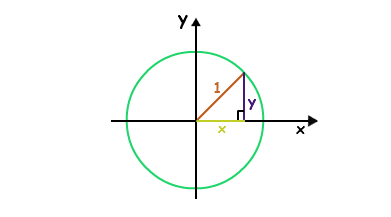# Unit Circle Formula

The unit circle formula has been explained here along with a solved example question. To recall, in mathematics, a unit circle is a circle with a radius of one. Especially in trigonometry, the unit circle is the circle of radius one centered at the origin (0, 0) in the Cartesian coordinate system in the Euclidean plane.

The unit circle is often denoted S1 while the generalization to higher dimensions is the unit sphere. The interior of the unit circle is called the open unit disk while the interior of the unit circle combined with the unit circle itself is called the closed unit disk.## Formula for Unit Circle

The general equation of circle is given below:

$\large \left(x-h\right)^{2}+\left(y-k\right)^{2}=r^{2}$

Where (h, k) are center coordinates and r is the radius.

### The unit circle formula is:

Unit Circle Formula (Equation) x2+y2=1

Where x and y are the coordinate values.

### Solved Examples on Unit Circle Formula

Question: Show that the point

$$\begin{array}{l}P\left[\frac{\sqrt{3}}{3},\,\frac{\sqrt{2}}{\sqrt{3}}\right]\end{array}$$
is on the unit circle.

Solution:

We need to show that this point satisfies the equation of the unit circle, that is:

$$\begin{array}{l}x^{2}+y^{2}=1\end{array}$$
$$\begin{array}{l}\left[\frac{\sqrt{3}}{3}\right]^{2}+\left[\frac{\sqrt{2}}{\sqrt{3}}\right]^{2}\end{array}$$
$$\begin{array}{l}=\frac{3}{9}+\frac{2}{3}\end{array}$$
$$\begin{array}{l}=\frac{1}{3}+\frac{2}{3}\end{array}$$

= 1

Therefore P is on the unit circle.